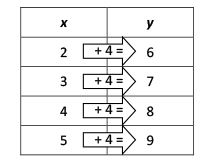Multiplicative Relationship

My Definition

Key Characteristics

The following are true of a multiplicative relationship:

• It is a relationship where two quantities can be expressed as multiples of each other.

• It can be generalized as y = ax, where y and x are multiples of each other and a reflects the scalar that is equivalent to the ratio of y to x.

Example

Video Transcript (PDF)

Non-exampleThis table shows an additive relationship between x and y, represented with
y = x + 4.

TEKS: 5(4)(C), 5(4)(D), 6(4)(A), 6(6)(C), 7(4)(C), 8(5)(A), 8(5)(E), 8(5)(F)